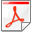Adaptive multiple subtraction using regularized nonstationary regressionNext: Introduction Up: Reproducible Documents

Adaptive multiple subtraction using regularized nonstationary regression

Sergey Fomel

Bureau of Economic Geology
John A. and Katherine G. Jackson School of Geosciences
The University of Texas at Austin
University Station, Box X
Austin, TX 78713-8924

Abstract:

Stationary regression is the backbone of different seismic data processing algorithms including match filtering, which is commonly applied for adaptive multiple subtraction. However, the assumption of stationarity is not always adequate for describing seismic signals. I present a general method of nonstationary regression and show its application to nonstationary match filtering. The key idea is the use of shaping regularization for constraining the variability of nonstationary regression coefficients.

As shown by simple computational experiments, shaping regularization has clear advantages over conventional Tikhonov's regularization, incuding a more intuitive selection of parameters and a faster iterative convergence.

Using benchmark synthetic data examples, I demonstrate successful applications of this method to the problem of adaptive subtraction of multiple reflections.Adaptive multiple subtraction using regularized nonstationary regressionNext: Introduction Up: Reproducible Documents

2013-07-26